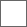### 孩子小学3年级数学题求教怎么做30*X+90*Y=210
X+Y=3200+10-90-30=一头大象tera是款好游戏，对于那些无脑喷我只想说：“爱玩玩，不爱玩请圆润地离开!”200+10=210
3头小象吃一天 30*3=90 所以不是3头小象
3头大象吃一天 90*3=270 所以也不是3头大象

200+10-30-90=90 剩下1头是大象200+10=210
90+90+90=270
90+90+30=210
90+30+30=150
30+30+30=90

XXX技术哪家强，广东东莞找你娘。

90x+30（3-x）-10=200
x=2

#### 扫二维码下载贴吧客户端